# How to Calculate EMA in Excel

Learn how to calculate the exponential moving average in Excel and VBA, and get a free web-connected spreadsheet.

The spreadsheet retrieves stock data from Yahoo Finance, calculates EMA (over your chosen time window) and plots the results.

The download link is at the bottom. The VBA can be viewed and edited; it’s completely free.

But first disover why EMA is important to technical traders and market analysts.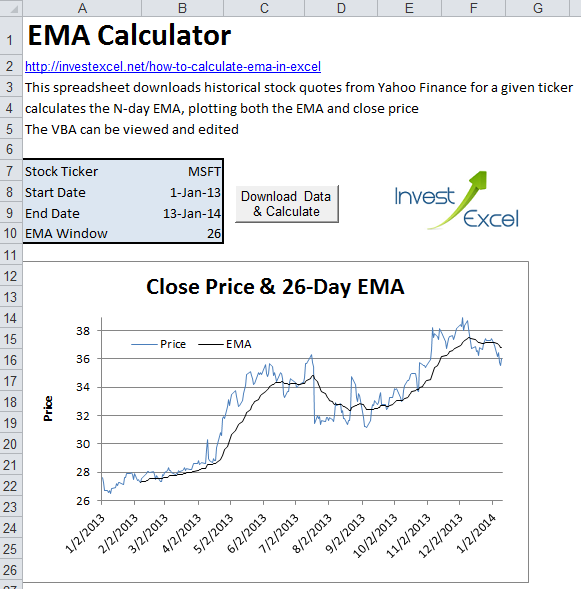Historical stock price charts are often polluted with a lot of high-frequency noise. This often obscures major trends. Moving averages help smooth out these minor fluctuations, giving you greater insight into the overall market direction.

The exponential moving average places greater importance on more recent data. The larger  the time period, the lower the importance of the most recent data.

EMA is defined by this equation.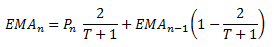where P is the price and T is the time period. Essentially, the EMA today is the sum of

• today’s price (multiplied by a weight)
• and yesterday’s EMA (multiplied by 1-weight)

You need to kickstart the EMA calculation with an initial EMA (EMA0). This is usually a simple moving average of length T.

The chart above, for example, gives the EMA of Microsoft between 1st January 2013 and 14th January 2014.

Technical traders often use the cross-over of two moving averages – one with a short timescale and another with a long timescale – to generate buy/sell signals. Often 12- and 26-day moving averages are used.

When the shorter moving average rises above the longer moving average, the market is trending updwards; this is a buy signal. However, when the shorter moving averages falls below the long moving average, the market is falling; this is a sell signal.

Let’s first learn how to calculate EMA using worksheet functions. After that we’ll discover how to use VBA to compute EMA (and automatically plot charts!)

## Calculate EMA in Excel with Worksheet Functions

Step 1. Let’s say that we want to calculate the 12-day EMA of Exxon Mobil’s stock price. We first need to get historic stock prices – you can do that with this bulk stock quote downloader.

Step 2. Calculate the simple average of the first 12 prices with Excel’s Average() function. In the screengrab below, in cell C16 we have the formula =AVERAGE(B5:B16) where B5:B16 contains the first 12 close prices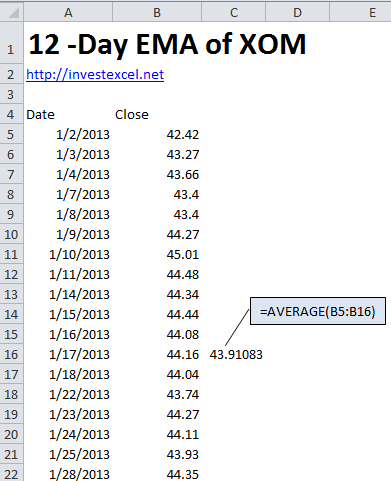Step 3. Just below the cell used in Step 2, enter the EMA formula above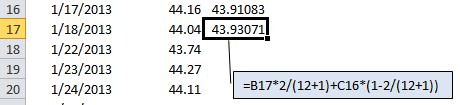Step 4. Copy the formula entered in Step 3 down to calculate the EMA of the entire set of stock prices.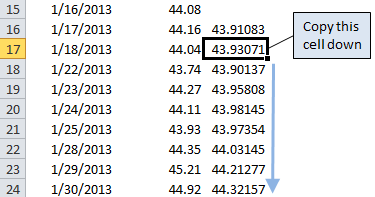There you have it! You’ve succesfully calculated an important technical indicator, EMA, in a spreadsheet.

## Calculate EMA with VBA

Now let’s mechanize the calculations with VBA, including the automatic creation of plots. I won’t show you the full VBA here (it’s available in the spreadsheet below), but we’ll discuss the most critical code.

Step 1. Download historical stock quotes for your ticker from Yahoo Finance (using CSV files), and load them into Excel or use the VBA in this spreadsheet to get historical quotes straight into Excel. Your data may look something like this: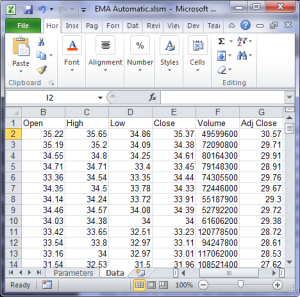Step 2. This is where we need to excercise a few braincells – we need to implement the EMA equation in VBA. We can use R1C1 style to programatically enter formulae into individual cells. Examine the code snippet below.

```1 2 Sheets("Data").Range("h" & EMAWindow + 1) = "=average(R[-" & EMAWindow - 1 & "]C[-3]:RC[-3])" Sheets("Data").Range("h" & EMAWindow + 2 & ":h" & numRows).FormulaR1C1 = "=RC[-3]*(2/(EMAWindow + 1)) + R[-1]C * (1-(2/(EMAWindow+1)))"```
• EMAWindow is a variable that equals the desired time window
• numRows is the total number of data points + 1 (the “+ 1” is because we’re assuming that the actual stock data starts on row 2)
• the EMA is calculated in column h

Assuming that EMAWindow = 5 and numrows = 100 (that is, there are 99 data points)

• the first line places a formula in cell h6 that calculates the arithmetic average of the first 5 historical data points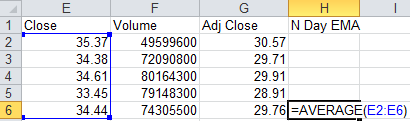• The second line places formulas in cells h7:h100 that calculates the EMA of the remaining 95 data points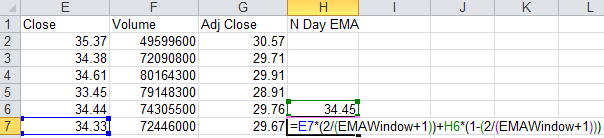Step 3
This VBA function creates a plot of the close price and EMA.

```1 2 3 4 5 6 7 8 9 10 11 12 13 14 15 16 17 18 19 20 21 22 23 24 25 26 27 28 29 30 31 Set EMAChart = ActiveSheet.ChartObjects.Add(Left:=Range("a12").Left, Width:=500, Top:=Range("a12").Top, Height:=300) With EMAChart.Chart   .Parent.Name = "EMA Chart"   With .SeriesCollection.NewSeries .ChartType = xlLine .Values = Sheets("data").Range("e2:e" & numRows) .XValues = Sheets("data").Range("a2:a" & numRows) .Format.Line.Weight = 1 .Name = "Price" End With   With .SeriesCollection.NewSeries .ChartType = xlLine .AxisGroup = xlPrimary .Values = Sheets("data").Range("h2:h" & numRows) .Name = "EMA" .Border.ColorIndex = 1 .Format.Line.Weight = 1 End With   .Axes(xlValue, xlPrimary).HasTitle = True .Axes(xlValue, xlPrimary).AxisTitle.Characters.Text = "Price" .Axes(xlValue, xlPrimary).MaximumScale = WorksheetFunction.Max(Sheets("Data").Range("e2:e" & numRows)) .Axes(xlValue, xlPrimary).MinimumScale = Int(WorksheetFunction.Min(Sheets("Data").Range("e2:e" & numRows))) .Legend.Position = xlLegendPositionRight .SetElement (msoElementChartTitleAboveChart) .ChartTitle.Text = "Close Price & " & EMAWindow & "-Day EMA"   End With```

Get this spreadsheet for the full working implementation of the EMA calculator with automatic download of historical data.

Download Excel Spreadsheet to Calculate EMA with VBA

## 16 thoughts on “How to Calculate EMA in Excel”

1. John Yard says:

Last time I downloaded one of your Excel speadsheets it caused my antivirus program to
flag it as a PUP (potential unwanted program ) in that apparently there was code imbedded in the download that was adware, spyware or at least potential malware.

It took literally days to clean up my pc. How can I ensure that I only download the Excel ?
Unfortunately there is incredible amounts of malware , adware and spywar, and you can’t be too careful.

If it is a question of cost I would not be unwilling to pay a reasonable sum, but the code must be PUP free. Thanks,

John Yard

1. There are no viruses, malware or adware in my spreadsheets. I’ve programmed them myself and I know exactly what’s inside them. There’s a direct download link to a zip file at the bottom of each point (in dark blue, bold and underlined). That’s what you should download. Hover over the link, and you should see a direct link to the zip file.

2. Mal says:

Hi Samir,

I want to use my access to live prices to create live tech indicators (ie RSI, MACD etc).

I have just realised in order for complete accuracy i need 250+ days worth of data for each stock as opposed to the 40 I have now.

Is there anywhere to access historical data of things like EMA, Avg Gain, Avg Loss that way I could just use that more accurate data in my model? Instead of using 252 days of data to get the correct 14 day RSI I could just get an externally sourced value for Avg Gain and Avg Loss and go from there?

I want my model to show results from 200+ stocks as opposed to a few.

Cheers

Mal

3. Sid says:

I want to plot multiple EMAs / BB / RSI on same chart and based on conditions would like to trigger trade. This would work for me as a sample excel backtester. Can you help me plot multiple timeseries on a same chart using same Data set.

Cheers

Sid

4. Mark says:

I know how to apply the raw data to an excel spreadsheet but how do you apply the ema results.
The ema in excel charts can’t be adjusted to specific periods.
Thanks

5. kliff mendes says:

Hi there Samir ,
Firstly thanks a million for all your hard work..outstanding job GOD BLESS . I just wanted to know if I have two ema plotted on chart lets say 20ema and 50ema when they cross either up or down can the word BUY or SELL appear at the cross over point will help me greatly ..
kliff mendes
texas

1. I’m working on a simple backtesting spreadsheet that’ll generate buy-sell signals. Give me some time…

6. Rajiv says:

Do you have any sheet in excel for 3 & 15 ema .

1. 3 and 15 period EMAs? You can do that easily with the spreadsheet and VBA in the article.

7. Khalid says:

Hello Mr. Samir ,
Firstly thanks a lot for the hard work you’re doing.
I am looking for the same work you did of EMA but with another Moving Average called TSMA (Time series M.A.)
Would you please do the same work for TSMA.

Thank you

8. Alpesh B Shah says:

Dear Mr. Samir,

Can you please guide me which is the best EMA value which provide correct buy or sell signal. I have used 12 as shown in your formulae.

Thanks

9. Gary says:

hi Samir !!

Great job on charts and explanations.

I have a question though. If I change the start date to a year later and look at recent EMA data, it is noticeably different than when I use the same EMA period with an earlier start date for the same recent date reference.

Is that what you expect ? It makes it difficult to look at published charts with EMAs shown and not see the same chart.

Thanks,

Gary

10. Shivashish Sarkar says:

Hi,
I am using your EMA calculator and I really appreciate. However, I have noticed that the calculator is not able to plot the graphs for all companies (it shows Run time error 1004). Can you please create an updated edition of your calculator in which new companies will be included??

11. Snehal vankudre says:

EXCELLENT DETAILED EXPLANATION. I wanted to know is there a formula to fetch direct EMA from yahoo or google finance, without downloading all the open and close stock data and then use formula of EMA. To be more precise, i want one liner data of EMA without getting historical data of stock. Pls guide.

1. No there isn’t. You’ll need to put some effort in